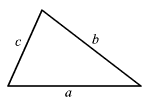# Triangle Inequality Theorem

The sum of the lengths of any two sides of a triangle is greater than the length of the third side.In the figure, the following inequalities hold.

$a+b>c$

$a+c>b$

$b+c>a$

Example 1:

Check whether it is possible to have a triangle with the given side lengths.

$7,9,13$

Add any two sides and see if it is greater than the other side.

The sum of $7$ and $9$ is $16$ and $16$ is greater than $13$ .

The sum of $9$ and $13$ is $21$ and $21$ is greater than $7$ .

The sum of $7$ and $13$ is $20$ and $20$ is greater than $9$ .

This set of side lengths satisfies the Triangle Inequality Theorem.

These lengths do form a triangle.

Example 2:

Check whether the given side lengths form a triangle.

$4,8,15$

Check whether the sides satisfy the Triangle Inequality Theorem.

Add any two sides and see if it is greater than the other side.

The sum of $4$ and $8$ is $12$ and $12$ is less than $15$ .

This set of side lengths does not satisfy Triangle Inequality Theorem.

These lengths do not form a triangle.